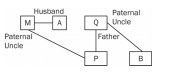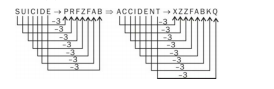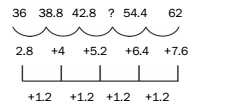# RRB ALP 2018 Practice Test Papers | Reasoning Questions (Day-21)

Dear Aspirants, Here we have given the Important RRB ALP & Technicians Exam 2018 Practice Test Papers. Candidates those who are preparing for RRB ALP 2018 can practice these Reasoning Questions to get more confidence to Crack RRB 2018 Examination.

[WpProQuiz 1784]

Click “Start Quiz” to attend these Questions and view Solutions

1. Which of the following is not correctly matched?
1. Basalt: Schist
2. Shale: Slate
3. Granite: Quartzite
4. Peat: Coal
1. Select the set of letters from the given alternatives which, when sequentially placed at the gaps in the given letter series, completes it. a_ab_a_aabb_aaabba_aabbaaa_bba
1. ababab
2. ababaa
3. abaaaa
4. aaaaaa
1. P’s father Q is B’s paternal uncle and A’s husband M is P’s paternal uncle. How is A related to B?
1. Mother
2. Sister
3. Daughter
4. None of these
1. Which of the following combinations of mathematical signs can correctly replace ‘*’ from

28 * 32 * 8 * 2 * 3 * 26?

1. +–×÷=
2. –+×÷=
3. +–÷×=
4. +÷–×=
1. Choose the odd one out.
1. 852
2. 529
3. 784
4. 841
1. 14: 9:: 26:?
1. 12
2. 13
3. 31
4. 15
1. 20, 38, 74, 146, 290,?
1. 576
2. 578
3. 580
4. 574
1. Choose the odd one out.
1. Binocular
2. Mirror
3. Magnifier
4. Microscope
1. In a certain coding system, if SUICIDE is coded as PRFZFAB, what will be the code for ACCIDENT?
1. XYYFABKQ
2. XZZFABJR
3. XZZFABKQ
4. ZZZFABKQ
1. 36 38.8 42.8? 54.4 62
1. 46
2. 48
3. 45
4. 48.2

Due to the effects of heat and pressure, igneous and sedimentary rocks change into metamorphic rocks. Granite converts into gneiss.

The given series is formed by repeating the set ‘aaabba’. So, option 4 will complete the series.As, P’s father = Q = B’s paternal uncle

A’s husband = M = P’s paternal uncle

Here, no actual relationship can be defined between A and B.

28 + 32 ÷ 8 – 2 × 3 = 28 + 4 – 6 = 28 – 2 = 26

All others are perfect squares, except 852.

14 = (2 × 9 – 4)

26 = (2 × 15 – 4)

Therefore, ? = 15

The pattern of the given series is as follows:

(20 × 2) – 2 = 38

(38 × 2) – 2 = 74

(74 × 2) – 2 = 146

(146 × 2) – 2 = 290

(290 × 2) – 2 = 578

All others magnify the image of an object, except mirror.The solution of the series is as follows:The number in place of?should be: 42.8 + 5.2 = 48.

RRB ALP 2018 Practice Test Papers | Reasoning Questions (Day-1)

RRB ALP 2018 Practice Test Papers | Reasoning Questions (Day-2)

RRB ALP 2018 Practice Test Papers | Reasoning Questions (Day-3)

RRB ALP 2018 Practice Test Papers | Reasoning Questions (Day-4)

RRB ALP 2018 Practice Test Papers | Reasoning Questions (Day-5)

RRB ALP 2018 Practice Test Papers | Reasoning Questions (Day-6)

RRB ALP 2018 Practice Test Papers | Reasoning Questions (Day-7)

RRB ALP 2018 Practice Test Papers | Reasoning Questions (Day-8)

RRB ALP 2018 Practice Test Papers | Reasoning Questions (Day-9)

RRB ALP 2018 Practice Test Papers | Reasoning Questions (Day-10)

RRB ALP 2018 Practice Test Papers | Reasoning Questions (Day-11)

RRB ALP 2018 Practice Test Papers | Reasoning Questions (Day-12)

RRB ALP 2018 Practice Test Papers | Reasoning Questions (Day-13)

RRB ALP 2018 Practice Test Papers | Reasoning Questions (Day-14)

RRB ALP 2018 Practice Test Papers | Reasoning Questions (Day-15)

RRB ALP 2018 Practice Test Papers | Reasoning Questions (Day-16)

RRB ALP 2018 Practice Test Papers | Reasoning Questions (Day-17)

RRB ALP 2018 Practice Test Papers | Reasoning Questions (Day-18)

RRB ALP 2018 Practice Test Papers | Reasoning Questions (Day-19)

RRB ALP 2018 Practice Test Papers | Reasoning Questions (Day-20)